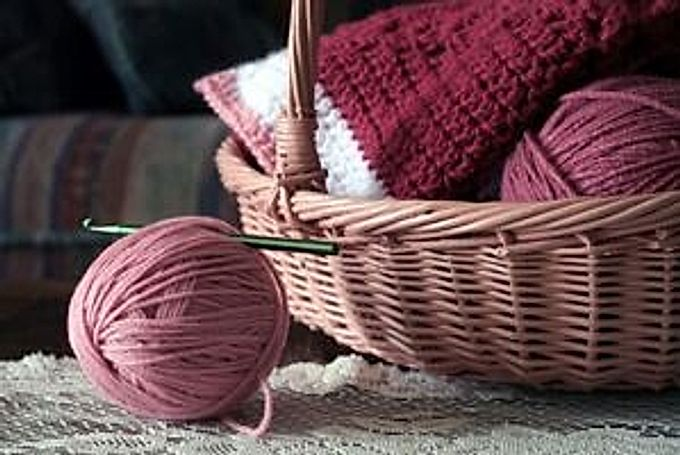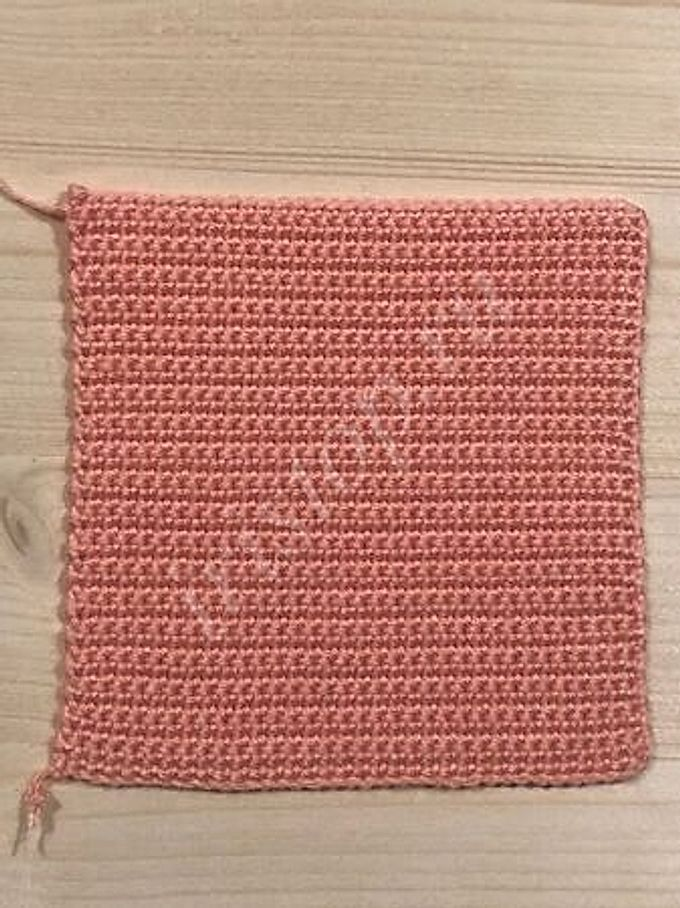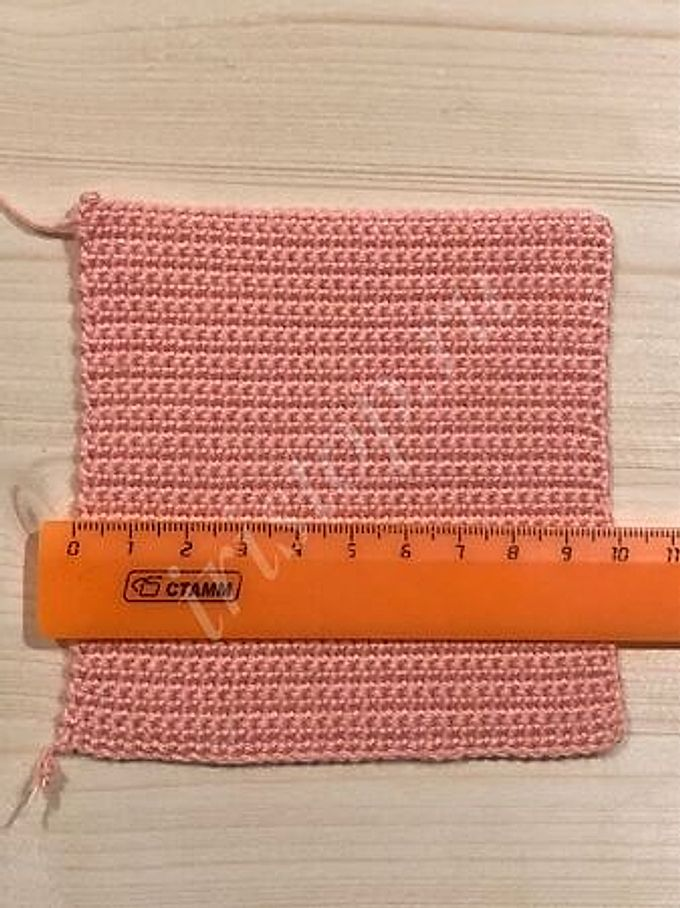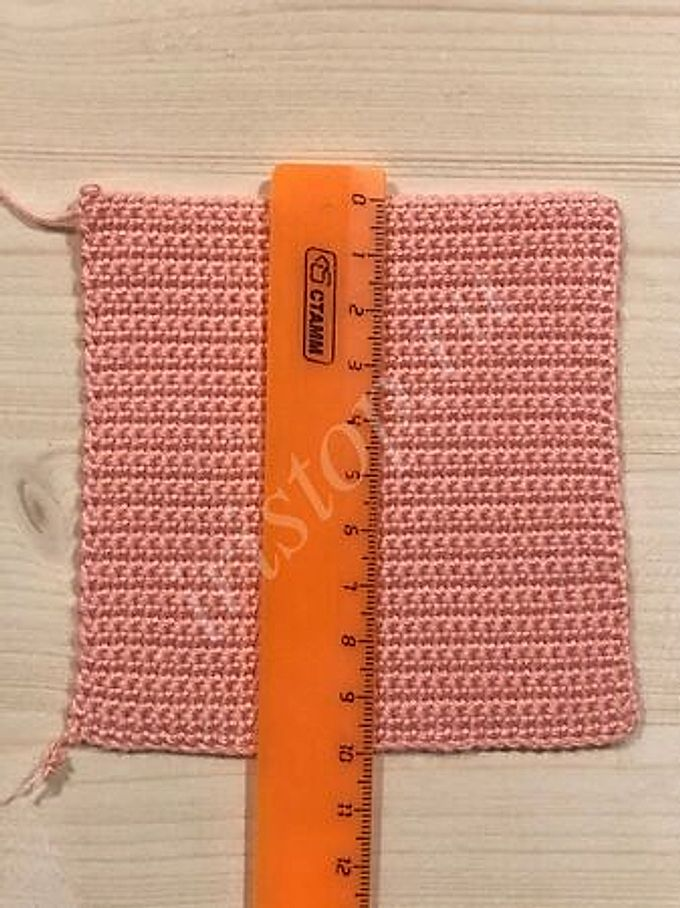# How to calculate crochet stitches

Natalie
Mon Jul 19 2021## Why do you need to calculate the loops

In this article I will tell you how to determine the number of loops and calculate the knitting density. To do this, we knit a sample. Note the pattern is knitted from the yarn of the future product and crocheted to be used when knitting.

My sample is knitted from Alize Diva yarn with single crochet, crochet number 2. The width and height of my sample is 10 cm.After that, the sample must be washed and ironed. It is such a sample that will show clear values ​​and help to calculate the number of loops that you need for your chosen product.

## How to calculate loops

Before we start calculating the number of loops, we need to determine the knitting density from the pattern that we prepared.

When knitting a 10 x 10 cm pattern, you need to count the number of air loops that you have scored. When I set my sample size 10 x 10, 35 air loops turned out, this number must be recorded.

Next, we will determine the number of loops that goes in 1 cm.

We take the number of loops in my case, this is 35 and divide by the width of the sample, that is, 3.5 loops are obtained by 10 cm. The main thing is that when calculating loops of 1 cm, if a fractional number is obtained (for example, 3.5), in no case should this number be rounded, since this number gives the total value when recruiting loops. That is, what figure turned out, that you leave. Thus, we have determined the number of loops that is included in 1 cm.

Next, we proceed to the calculation of the loops required for the selected product.

To do this, you need to know the width of the product. We multiply the width of the product by the number of loops in 1 cm.

For example, the width of the product is 29 cm, we multiply by 3.5 loops (the number of loops in 1 cm) and we get 101.5 loops. When we have determined the number of loops, the resulting figure is rounded to 101 or to 102 loops.

If you knit a product in which the first row is knitted with double crochets, then add 3 loops to your value. For example 102 stitches + 3 stitches = 105 stitches. These 3 stitches represent lifting stitches, which we type at the beginning of the row before starting to knit a double crochet.

But do not forget that this is only necessary when crocheting double crochets. If you knit in single crochets, you leave your resulting number, in my case it is 102 loops.